# CSS3 圖像取值與生成內容模組

(Redirected from Css3-images)

## 圖像取值 ― <image> 型態

```<image> = <url> | <image-list> | <element-reference>  | <gradient>
```

### 後備圖像與註解 ― ‘`image()`’ 表示法

`image()`’ 表示法的定義為：

```<image-list> = image( [ <image-decl> , ]* [ <image-decl> | <color> ] )
<image-decl> = <string> [ ltr | rtl ]?
```

### 把元素當作圖像使用 ― ‘`element()`’ 表示法

`element()`’ 的語法為：

```<element-reference> = element( <id-selector> )
```

## 漸層

• `background: linear-gradient(white, gray);`
• `list-style-image: radial-gradient(circle, #006, #00a 90%, #0000af 100%, white 100%)`

### 線性漸層 ― ‘`linear-gradient()`’ 表示法

```<linear-gradient> = linear-gradient(
[ [ <angle> | to <side-or-corner> ] ,]?
<color-stop>[, <color-stop> ]+
)

<side-or-corner> = [ left | right ] || [ top | bottom ]
```

`270deg` ’。#### 線性漸層實例

```linear-gradient(yellow, blue);
linear-gradient(to bottom, yellow 0%, blue 100%);
```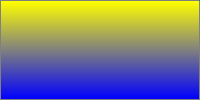```linear-gradient(135deg, yellow, blue);
```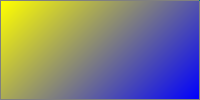```linear-gradient(yellow, blue 20%, #0f0);
```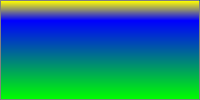```linear-gradient(to top right, red, white, blue)
```
（SVG 圖片）

### 放射漸層 ― ‘`radial-gradients()`’ 表示法

```<radial-gradient> = radial-gradient(
[ [ <shape> || <size> ] [ at <position> ]? , |
at <position>,
]?
<color-stop> [ , <color-stop> ]+
)
```

```radial-gradient(5em circle at top left, yellow, blue)
```

<position>

<shape>

<size>

`circle`’ 漸層與 ‘`ellipse`’ 漸層都接受下面的關鍵字作為 <size>

`closest-side`

`farthest-side`

`closest-corner`

`farthest-corner`

<length>

[ <length> | <percentage> ]{2}

```<radial-gradient> = radial-gradient(
[ [ circle               || <length> ]                          [ at <position> ]? , |
[ ellipse              || [ <length> | <percentage> ]{2} ]    [ at <position> ]? , |
[ [ circle | ellipse ] || <extent-keyword> ]                  [ at <position> ]? , |
at <position> ,
]?
<color-stop> [ , <color-stop> ]+
)
<extent-keyword> = closest-corner | closest-side | farthest-corner | farthest-side
```

#### 放射漸層範例

```radial-gradient(yellow, green);
```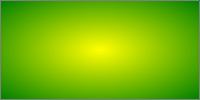```radial-gradient(circle, yellow, green);
```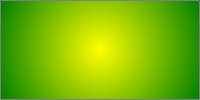```radial-gradient(red, yellow, green);
``````radial-gradient(farthest-side at left bottom, red, yellow 50px, green);
```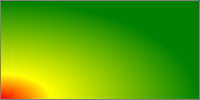```radial-gradient(closest-side at 20px 30px, red, yellow, green);
``````radial-gradient(closest-side circle at 20px 30px, red, yellow, green);
```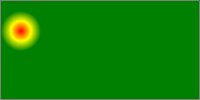### 漸層顏色站點

```<color-stop> = <color> [ <percentage> | <length> ]?
```